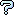All about flooble | fun stuff | Get a free chatterbox | Free JavaScript | Avatarsperplexus dot infoCut Wire (Posted on 2005-05-20)A piece of wire is to be cut into two pieces (one bent into the shape of a regular p-gon and the other a regular q-gon).

If

1) p = 2*q,
2) 2*perimeter(q-gon) = 3*perimeter(p-gon), and
3) the sum of the two areas is minimized;

then what is the value of q?

 See The Solution Submitted by Bractals No RatingComments: ( Back to comment list | You must be logged in to post comments.)re-interpretation Comment 6 of 6 |From Bractals' comment, I am wondering if he meant condition 2 to be a variable.   i.e.:

Let x= the fraction of wire in the p-gon   (0 <= x <= 1)
So x is a variable instead of being fixed at .4
For different values of q, use calculus to minimize the total area by varying x.
See how x is related to q
For what value of q is x closest to .4

If this is Bractals' intent, then my intuition tells me that as q approaches infinity, (circles), the optimum x approaches .5;
and x will be closest to .4 when q=3

Edited on May 21, 2005, 7:20 pm
 Posted by Larry on 2005-05-21 19:10:30Please log in:

 Search: Search body:
Forums (0)